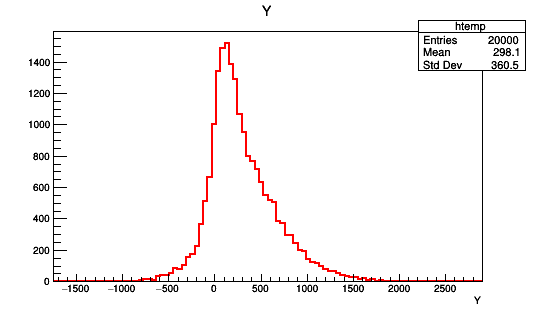# How to simply make a histogram from .dat file

_ROOT Version:ROOT 6.12/06
_Platform:Debian?
_Compiler:

I have a .dat file, it is separated in 2 columns and about 20 000 rows.
I want to open this file somehow and plot a histogram for one of the columns.

I have looked at these two threads:

and tried this code but it does not work for me.

I understand how to draw a histogram based off of things that I have calculated (example: write a code to calculate the energy then make a histogram of this energy)

I just don’t know what command to use to read my .dat file and make the histogram

I am completely new to c++ and root

Hi,

can you attach the first 10 or 20 lines of the file or share it entirely so that I can produce an example which works?

Best,
D

So here there is a number between 1 and 5, and then another value next to it.

Right now I want to plot the other value, regardless of whether it is 1,2,3,4,5

I converted to a .txt file and attached. I assume the code is the same for .txt and .dat? It won’t let me attach .datevents.txt (292.2 KB)

The code shown above works just fine with your file.

So what do you mean exactly with “it does not work for me.”?Which code then? For me, root just kept “crashing” and nothing would run.

I also want to know how to choose certain elements to plot.

Let’s say I would want to plot only the numbers that are with 1, how would I do it ?

tree->Draw(“x”);
I guess I don’t know how to write the file name in a way that works, since it doesn’t accept it like this. Also don’t know what “draw(x)” will be drawing (first column, etc)

or if I need to change x,y,z

``````TTree *t = new TTree("t", "my events");
t->Print();
t->Draw("first");
``````

ah, I see. Thank you.

I have another question, if I wanted to select to plot only the energies corresponding to 1, or to 2 etc how would I arrange this ?

``````t->Draw("second", "first == 1");
``````

Hi,

this is the modern way to achieve this:

``````auto df = ROOT::Experimental::TDF::MakeCsvDataFrame("data.txt", false, ' ');
auto h = df.Histo1D("Col1")
h->Draw();
``````

It has plenty of advantages, besides being the suggested way to code:

1. You can easily convert your dataset to ROOT:
``````auto df = ROOT::Experimental::TDF::MakeCsvDataFrame("data.txt", false, ' ');
df.Snapshot("data", "data.root"); // <- one line is enough
auto h = df.Histo1D("Col1")
h->Draw();
``````
1. You can run using all the cores of your machine (not mutually exclusive with 1.)
``````ROOT::EnableImplicitMT(); // <- just adding this line is enough
auto df = ROOT::Experimental::TDF::MakeCsvDataFrame("data.txt", false, ' ');
df.Snapshot("data", "data.root");
auto h = df.Histo1D("Col1")
h->Draw();
``````
1. You can create several histograms in the same event loop, therewith limiting the disk access:
``````ROOT::EnableImplicitMT(); // <- just adding this line is enough
auto df = ROOT::Experimental::TDF::MakeCsvDataFrame("data.txt", false, ' ');
df.Snapshot("data", "data.root");
auto h = df.Histo1D("Col1");
auto h1 = df.Histo1D("Col0");
auto hWeighted = df.Histo1D("Col1", "Col0");
auto h2 = df.Histo2D("Col0", "Col1");
// ...
``````

See all different options in these examples.

Best,
D

One last thing, if I wanted to make a 2D TH2F distribution, how would I proceed ?

Hi,

it’s in my point 2):

``````auto h2 = df.Histo2D("Col0", "Col1");
``````

Cheers,
D

When I run it for the 1D, I simply get a blank canvas. When I run with this line for the 2D, I get many errors

error: no matching member function for call to ‘Histo2D’

among other things.

Sorry if these questions are basic, I am very new to this

Hi,

these lines have been tested on my machine and I am sure they work. I think we are missing something fundamentalCan you share in a post the code which leads to the errors you are reporting? I am sure we can solve those quickly.

Cheers,
D

``````void two_d()
{
auto df = ROOT::Experimental::TDF::MakeCsvDataFrame("events.dat", false, ' ');
auto h2 = df.Histo2D("Col0", "Col1");
h2->Draw();

}
``````

I really don’t know what to add or to write

Hi,

can you try:

``````      auto df = ROOT::Experimental::TDF::MakeCsvDataFrame( "data.txt", false, ' ');
auto h = df.Histo2D({"myHisto", "My Title", 5, 0.5, 5.5, 256, -400, 400}, "Col0", "Col1");
h->Draw();
``````

Cheers,
Danilo

This is what I get

Processing Histo2D.C…
terminate called after throwing an instance of ‘std::runtime_error’
what(): Unknown columns: Col0,Col1

Now it runs, but it only produces a blank canvas c1

Ok.
This is a well known behaviour independent of data frame. You need to make sure the object survives after the end of the scope. For example:

``````     auto df = ROOT::Experimental::TDF::MakeCsvDataFrame( "data.txt", false, ' ');
auto h = df.Histo2D({"myHisto", "My Title", 5, 0.5, 5.5, 256, -400, 400}, "Col0", "Col1");
auto mycanvas = new TCanvas();
h->DrawCopy();
``````

This works, but it produces a result not like I had expected. Glad to see it working though. Thanks for your help !